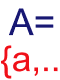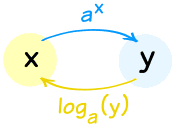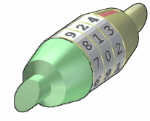# Algebra 2

Also known as "College Algebra"

OK. So what are you going to learn here?

You will learn about Numbers, Polynomials, Inequalities, Sequences and Sums, many types of Functions, and how to solve them.

You will also gain a deeper insight into Mathematics, get to practice using your new skills with lots of examples and questions, and generally improve your mind.

With your new skills you will be able to put together mathematical models so you can find good quality solutions to many tricky real world situations.

Near the end of most pages is a "Your Turn" section ... do these! You need to balance your reading with doing. Answering questions helps you sort things out in your mind. And don't guess the answer: use pen and paper and try your best before seeing the solution.

## Language

So what is this thing called Mathematics? And how do you go about learning it?## Sets

Next, we need to think about mathematics in terms of sets.## Numbers

Now we know what a set is, let us look at different sets of numbers that are useful:## Inequalities

"Equal To" is nice but not always available. Maybe we only know that something is less than, or greater than. So let's learn about inequalities.

a≥b

## Exponents

We will be using exponents a lot, so let's get to know them well.## Polynomials

Polynomials were some of the first things ever studied in Algebra. They are simple, yet powerful in their ability to model real world situations.## Equations

And, of course, we need to know about equations ... and how to solve them.

## Graphs

Graphs can save us! They are a great way to see what is going on and can help us solve many things. But we need to be careful, as they sometimes don't give the full story.## Linear Equations

They are just equations for lines. But they come in many forms.## Functions

A function relates an input to an output. But from that simple foundation many useful things can be built.## Equations of Second Degree

"Second degree" just means the variable has an exponent of 2, like x2. It is the next major step after linear equations (where the exponent is 1, like x).## Solving## Solving Inequalities

We learned about inequalities above, now let's learn how to solve them.

## Exponents and Logarithms

We already know about exponents ... well logarithms just go the other way. And together they can be very powerful.## Systems of Linear Equations

What happens when we have two or more linear equations that work together? They can often be solved! It isn't very hard but can take a lot of calculations.## ProbabilityIs it likely? You be the judge!

## Sequences, Series and Partial Sums

A Sequence is a set of things (usually numbers) that are in order. We can also sum up a series, where Sigma Notation is very useful.## Finally

These last few subjects use what we have learned above.

And that is all!

But there are many other interesting algebra topics such as: